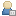###Show Posts

This section allows you to view all posts made by this member. Note that you can only see posts made in areas you currently have access to.

### Messages - heesch

Pages: 
1
##### RNA structures (DSSR) / Re: Circular DNA parameters
« on: April 14, 2021, 06:23:14 am »
Dear Xiang-Jun,

That looks very pretty!!! Thank you very much for the follow-up!

Some while ago I managed to cook-up a "solution" using python by adjusting some of the elegant x3DNA parameters.
Sorry for not sharing my solution any sooner!

My approach assumed that every 10 basepairs one should adjust the roll angle to obtain a circle.
However, for sequences that have a length that is not a 10 fold I ran into issues, creating super helices...
So I randomly distributed the "remaining" basepairs among chunks of 10bp and adjusted the twist angle accordingly.

In the future I might work on building super coils!
Although, I have not had the time nor leads on how to do itSo if anybody has some suggestions or ideas, I would be more than happy to hear them!

Here a snippet of python (v3) code to determine the new roll and twist parameters...
Code: [Select]
`        import random         import numpy as np        n_bp = len(sequence)              remainder = (n_bp % 10)        if remainder != 0:            raw_turns = n_bp / 10            turns = int((n_bp-remainder) / 10)            turn_angle = 360/turns            bp_angle =  360/n_bp            turn_indices = list(np.arange(0,n_bp,10))            selection = sorted(random.sample(turn_indices[1:], remainder))            count = 0            new_turn_indices = []            for index in turn_indices:                if index in selection:                    count +=1                    new_turn_indices.append(index+count)                else:                    new_turn_indices.append(index+count)            differences = [new_turn_indices[n]-new_turn_indices[n-1] for n in range(1,len(new_turn_indices))]            turn_angles = [diff*bp_angle for diff in differences]            count = 0            roll_angles = []            for i in range(0,n_bp):                if i in new_turn_indices:                    roll_angles.append(turn_angles[count])                    count+=1                else:                    roll_angles.append(0)            new_twist = 360/11            twist = 360/10            twists = []            for diff in differences:                if diff == 10:                    twists.extend([twist]*diff)                else:                    twists.extend([new_twist]*diff) `

2
##### RNA structures (DSSR) / Circular DNA parameters
« on: October 30, 2020, 01:27:01 pm »
Dear Dr. Xiang-Jun Lu,

Thank you for developing (and maintaining) this collection of beautiful and practical DNA geometry software!

For my project I want to generate circular DNA (plasmids) of various sizes.
Based on the book chapter of Calladine et al. (see source below) I found a way to determine the base roll angle for each base pair.

For example, we can determine the curvature of each helical turn to make a circle consisting of 100 bp as follows:

- assume 10 bp per turn
- assume helix angle of 36 degrees

First determine number of turns: 10
Determine the angle each turn has to make: 36 degrees
For a smooth transition per base step fit this angle to cosine:

First we need to determine the amplitude to account for +/- direction of helix
amplitude = 36 / (10*0.5)

Then each roll angle can be determined as follows:
for bp_i in range[0:100]:
roll_angle = amplitude*(cos(helix_angle)*bp_i))

which gives you periodic values of:
[7.2, 5.825, 2.225, -2.225, -5.825, -7.2, -5.825, -2.225,  2.225, 5.825, ....]

Of course this (could) work for an arbitrary length of sequences.
So may plan was to use these roll angle parameters to make circular DNA.

As a starting point I just used for each bp a twist and rise value of 36 and 3.34 respectively (since I want B-DNA) together with the periodic roll angle values and all other parameters black (zero). See attachement for parameter file.

When I visualize the structure with the rebuilder at the webserver (http://web.x3dna.org/custom/option) the result is very close to perfect, see image in attachement. However, the ring is not perfectly planar (although I want to have the geometry completely planar such that I can easily define the connections of the beginning and end in the pdb later on).

I very much hope that with your years of DNA geometry experience you could provide some insight on how to make the ring perfectly planar. I am probably overlooking something obvious... So apologies in advance if the question is unclear!

Thank you for reading my message and stay safe!

Best,
Thor

PS
Eventually I want to make this to work for arbitrary length of sequences, however, conceptually I couldn't think of a smart way to deal with the decimal numbers arising in the total number of "complete" turns. I guess one should start to introduce varying helix twists such that the outer ends properly meet?

Source: Calladine, C.R., Drew, H.R., Luisi, B.F. & Travers, A.A. Understanding DNA: theMolecule and How It WorksChapter 4 (Elsevier Academic Press, San Diego, CA,2004).

Pages: 

Created and maintained by Dr. Xiang-Jun Lu [律祥俊] (xiangjun@x3dna.org)
The Bussemaker Laboratory at the Department of Biological Sciences, Columbia University.